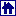You are reading help file online using chmlib.comInvoice Template Variables

 INVOICE \STATUS\ Invoice status (For example: Expected within 30 days, Settled 5 days earlier etc.) \DATE_DUE\ \SETTLEMENT_DATE\ \DUE_DATE\ Date when the Invoice is due in following format: 10/4/2006. \DATE_DUE_LONG\ \SETTLEMENT_LONGDATE\ \DUE_DATELONG\ Date whenthe Invoice is due in following format: Monday, October 04, 2006. \INVOICE_DATE\ \INV_DATE\ Date the Invoice was sent in following format: 10/4/2006. \INVOICE_DATE_LONG\ \INV_LONGDATE\ Date the Invoice was sent in following format: Monday, October 04, 2006. \INVOICE _CODE\ \INV_CODE\ Invoice code \INVOICE _GLOBAL_CODE\ \INV_GLOBAL\ \INV_GLOBALLONG\ Invoice global code. \INVOICE_TOTAL\ \INV_TOTAL\ Invoice total. \JOBS_TOTAL\ \JOBSTOTAL\ Jobs total. \TAX1\ \TAX\ Used in algorithms (If Tax1 is set = True, if it does not = False) \TAX1_NAME\ \TAX1NAME\ \TAXNAME\ Tax1 name. \TAX1_VALUE\ \TAX1VALUE\ \TAXVALUE\ Tax1 value. \TAX2\ Used in algorithms (If Tax2 is set = True, if it does not = False) \TAX2_NAME\ \TAX2NAME\ Tax2 name. \TAX2_VALUE\ \TAX2VALUE\ Tax2 value. \DISCOUNT1\ \DISCOUNT\ Used in algorithms (If Discount1 is set = True, if it does not = False) \DISCOUNT1_NAME\ \DISCOUNTNAME\ \DISCOUNT1NAME\ Discount1 name. \DISCOUNT1_VALUE\ \DISCOUNT1VALUE\ \DISCOUNTVALUE\ Discount1 value. \DISCOUNT2\ Used in algorithms (If Discount2 is set = True, if it does not = False) \DISCOUNT2_NAME\ \DISCOUNT2NAME\ Discount2 name. \DISCOUNT2_VALUE\ \DISCOUNTVALUE\ Discount2 value. \TAXES\ Used in algorithms (If any tax is set = True, if it they are not = False) \DISCOUNTS\ Used in algorithms (If discounts exist = True, if there are no = False) \AFTER_DISCOUNT1\ \AFTERDISCOUNT1\ \AFTERDISCOUNT\ Total after Discount1 applied. \NET_JOBS_TOTAL\ \NETJOBSTOTAL\ Jobs total with discounts applied. \AFTER_TAX1\ \AFTERTAX1\ Total after Tax1 applied. \INV_DUE\ \INVOICE_DUE\ Balance due. \INV_PAID\ \INVOICE_PAID\ Sum paid by this Invoice. \INV_IS_PAID\ Used in algorithms (If the Invoice is paid = True, if its not = False) \INVOICE_PAYMETHOD\ \PAYMETHOD\ Invoice Payment Method \INVOICE_PAYMETHOD_DESCR\     \PAYMETHOD_DESCR\ Invoice Payment Method Description \BEFORE_ADJUSTMENTS\ Invoice total excluding Adjustments \ADJUSTMENTS_VALUE\ Value of the Adjustments \ADJUSTMENTS_DESCR\ Description of the Adjustments

Dataset (Jobs added to the Invoice):